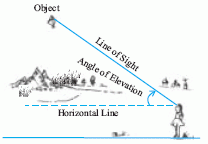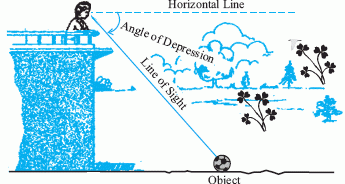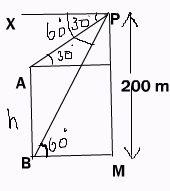Email us to get an instant 20% discount on highly effective K-12 Math & English kwizNET Programs!

#### Online Quiz (WorksheetABCD)

Questions Per Quiz = 2 4 6 8 10

### High School Mathematics - 210.2 Heights and Distances

 Introduction One of the most important applications of trigonometry is the application of measurement of heights and distances which cannot be measured directly. It is also extensively used by astronomers in determining the distance of the heavenly bodies like the sun and moon and stars. Two angles are very often used in the practical applications of trigonometry . Line of sight The line of object is the line from our eyes to object. Angle of Elevation If the object is above the horizontal level of our eyes, we have to turn our head upwards to view the object. This is called the angle of elevation.Angle of Depression If the object is below the horizontal level of our eyes, we have to bend our head to view it. This is called the angle of elevation.Example 1: The angle of elevation of the top of a tower at a distance of 100 metres from its foot on a horizontal plane is found to be 60o. Find the height of the tower. Let CA be the tower equal to h metres in length and B point at a distance of 100 metres from its foot C. It is given that angle ABC = 60oFrom the right angle triangle ABC we have h/100 = tan 60 Solving the above we get h = 173.2 metres Answer: Height of the tower is 173.2 metres Example 2: From the top of a cliff, 200 metres high, the angle of depression of the top and bottom of a tower are observed to be observed to be 30o and 60o, find the height of the tower.Solution: Let AB represent the tower and P the top of cliff MP. If PX be the horizontal line through P, then angle XPA = 30o and angle XPB = 60o. Let the height of the tower be h metres. From A draw AL perpendicular to PM. ML = AB = h=> LP = (200-h) Again, ang PBM = ang XPB = 60 ang PAL = ang XPA = 30 (alternate angles ) From the right triangle PMB, BM/200 = cot 60 BM = 200 cot 60 = 200/3 From the right triangle PLA, AL /AP = cot 30 =>AL = LP cot 30 = =�(200-h)3 But AL = BM = =�(200-h)3 =200/3 Answer: h = 133 1/3 metres Directions: Answer the following questions. Also write at least 10 examples of your own.
 Q 1: If the shadow of a tower is 30 metres, when the sun's altitude is 30o, what is the length of the shadow when the sun's altitude is 60o?240 metres100 metres200 metres Q 2: The angle of elevation of the top of a tower from a point 60m from its foot is 30o. Find the height of the tower.20 m2031/2 m100 m Q 3: What is the angle of elevation of the sun when the length of the shadow of a pole is 31/2 times the height of the pole?60o90o30o Q 4: A vertical flagstaff stands on a horizontal plane from a point distant 150 metres from its foot, the angle of elevation of its top is found to be 30o, find the height of the flagstaff.100 metres113.4 metres100.4 metres Q 5: The angular elevation of a tower from a point is 30 degrees at a point in a horizontal line to the foot of the tower and 100 metres near it is 60 degrees, find the distance of the first point from the tower. 100 metres86.6 metres150 metres Q 6: The elevation of a tower at a point 60 metres from it is cot-13/5 Obtain the height of the tower.45 metres110 metres100 metres Q 7: The horizontal distance between two towers is 60 metres and the angular depression of the top of the first as seen from the top of the second, which is 150 metres is 30o. Find the height of the first.115.36 metres120 metres100 metres Q 8: A person standing on the bank of a river, observes that the angle subtended by a tree on the opposite bank is 60 degrees, when he retires 40 metres from the bank, he finds the angle to be 30 degrees. Find the height of the tree.20 metres64 metres34 metres Question 9: This question is available to subscribers only! Question 10: This question is available to subscribers only!

#### Subscription to kwizNET Learning System offers the following benefits:

• Unrestricted access to grade appropriate lessons, quizzes, & printable worksheets
• Instant scoring of online quizzes
• Progress tracking and award certificates to keep your student motivated
• Unlimited practice with auto-generated 'WIZ MATH' quizzes